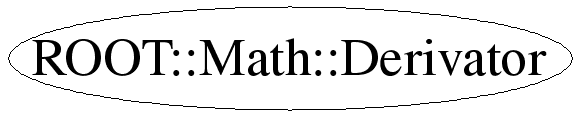# class ROOT::Math::Derivator

```
Class for computing numerical derivative of a function.
Presently this class is implemented only using the numerical derivatives
algorithms provided by GSL
using the implementation class ROOT::Math::GSLDerivator

This class does not support copying

@ingroup Deriv

```

## Function Members (Methods)

public:
 virtual ~Derivator() ROOT::Math::Derivator Derivator() ROOT::Math::Derivator Derivator(const ROOT::Math::IGenFunction& f) ROOT::Math::Derivator Derivator(const ROOT::Math::Derivator::GSLFuncPointer& f, void* p = 0) double Error() const double Eval(double x, double h = 1E-8) const static double Eval(const ROOT::Math::IGenFunction& f, double x, double h = 1E-8) static double Eval(const ROOT::Math::IMultiGenFunction& f, const double* x, unsigned int icoord = 0, double h = 1E-8) static double Eval(ROOT::Math::IParamFunction& f, double x, const double* p, unsigned int ipar = 0, double h = 1E-8) static double Eval(ROOT::Math::IParamMultiFunction& f, const double* x, const double* p, unsigned int ipar = 0, double h = 1E-8) double EvalBackward(double x, double h = 1E-8) const static double EvalBackward(const ROOT::Math::IGenFunction& f, double x, double h = 1E-8) double EvalCentral(double x, double h = 1E-8) const static double EvalCentral(const ROOT::Math::IGenFunction& f, double x, double h = 1E-8) double EvalForward(double x, double h = 1E-8) const static double EvalForward(const ROOT::Math::IGenFunction& f, double x, double h = 1E-8) double Result() const void SetFunction(const ROOT::Math::IGenFunction& f) void SetFunction(const ROOT::Math::Derivator::GSLFuncPointer& f, void* p = 0) int Status() const
private:
 ROOT::Math::Derivator Derivator(const ROOT::Math::Derivator&) ROOT::Math::Derivator& operator=(const ROOT::Math::Derivator&)

## Data Members

private:
 ROOT::Math::GSLDerivator* fDerivator

## Class Charts## Function documentation

```Empty Construct for a Derivator class
Need to set the function afterwards with Derivator::SetFunction

```
explicit Derivator(const IGenFunction &f)
```Construct using a GSL function pointer type
@param f :  free function pointer of the GSL required type
@param p :  pointer to the object carrying the function state
(for example the function object itself)

```
explicit Derivator(const ROOT::Math::Derivator::GSLFuncPointer& f, void* p = 0)
virtual ~Derivator()
``` destructor
```
Derivator(const Derivator &)
``` disable copying
```
void SetFunction(const ROOT::Math::IGenFunction& f)
```Template methods for generic functions
Set the function f for evaluating the derivative.
The function type must implement the assigment operator,
<em>  double  operator() (  double  x ) </em>

```
SetFunction(const ROOT::Math::Derivator::GSLFuncPointer& f, void* p = 0)
double Eval(double x, double h = 1E-8) const
```Computes the numerical derivative of a function f at a point x.
It uses Derivator::EvalCentral to compute the derivative using an
adaptive central difference algorithm with a step size h

```
double EvalCentral(double x, double h = 1E-8) const
```Computes the numerical derivative at a point x using an adaptive central
difference algorithm with a step size h.

```
double EvalForward(double x, double h = 1E-8) const
```Computes the numerical derivative at a point x using an adaptive forward
difference algorithm with a step size h.
The function is evaluated only at points greater than x and at x itself.

```
double EvalBackward(double x, double h = 1E-8) const
```Computes the numerical derivative at a point x using an adaptive backward
difference algorithm with a step size h.
The function is evaluated only at points less than x and at x itself.

```
double Eval(const ROOT::Math::IGenFunction& f, double x, double h = 1E-8)
``` @name --- Static methods ---
This methods don't require to use a Derivator object, and are designed to be used in
fast calculation. Error and status code cannot be retrieved in this case

Computes the numerical derivative of a function f at a point x.
It uses Derivator::EvalCentral to compute the derivative using an
adaptive central difference algorithm with a step size h

```
double EvalCentral(const ROOT::Math::IGenFunction& f, double x, double h = 1E-8)
```Computes the numerical derivative of a function f at a point x using an adaptive central
difference algorithm with a step size h

```
double EvalForward(const ROOT::Math::IGenFunction& f, double x, double h = 1E-8)
```Computes the numerical derivative of a function f at a point x using an adaptive forward
difference algorithm with a step size h.
The function is evaluated only at points greater than x and at x itself

```
double EvalBackward(const ROOT::Math::IGenFunction& f, double x, double h = 1E-8)
```Computes the numerical derivative of a function f at a point x using an adaptive backward
difference algorithm with a step size h.
The function is evaluated only at points less than x and at x itself

```
double Eval(const ROOT::Math::IMultiGenFunction& f, const double* x, unsigned int icoord = 0, double h = 1E-8)
``` Derivatives for multi-dimension functions

Evaluate the partial derivative of a multi-dim function
with respect coordinate x_icoord at the point x[]

```
double Eval(IParamFunction & f, double x, const double * p, unsigned int ipar = 0, double h = 1E-8)
```Evaluate the derivative with respect a parameter for one-dim parameteric function
at the point ( x,p[]) with respect the parameter p_ipar

```
double Eval(IParamMultiFunction & f, const double * x, const double * p, unsigned int ipar = 0, double h = 1E-8)
```Evaluate the derivative with respect a parameter for a multi-dim parameteric function
at the point ( x[],p[]) with respect the parameter p_ipar

```
int Status()
```return the error status of the last derivative calculation

```
double Result()
```return  the result of the last derivative calculation

```
double Error()
```return the estimate of the absolute error of the last derivative calculation

```

Last update: root/mathmore:\$Id: Derivator.h 21503 2007-12-19 17:34:54Z moneta \$
Copyright (c) 2004 ROOT Foundation, CERN/PH-SFT *

This page has been automatically generated. If you have any comments or suggestions about the page layout send a mail to ROOT support, or contact the developers with any questions or problems regarding ROOT.